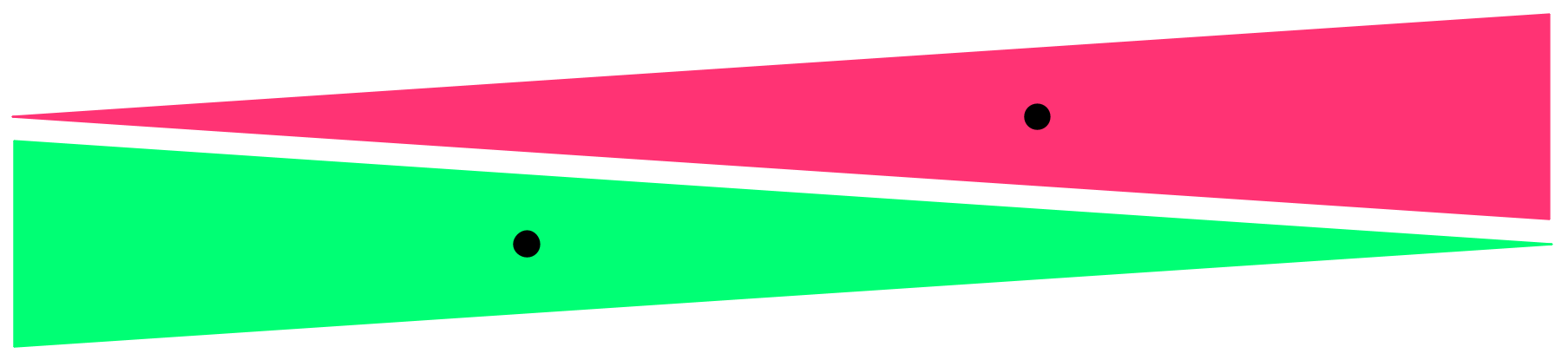# Continuity of barycentre in Hausdorff metric

Let $K_1$, $K_2$ be two convex compact sets in $\mathbb{R}^d$, and $p_1,p_2$ be their barycenters. Is it true that the distance between $p_1$ and $p_2$ does not exceed a Hausdorff distance between $K_1$ and $K_2$? If not, maybe there is some weaker estimate, say, uniform continuity of the map (convex body)$\rightarrow$ (its barycenter) for bodies inside, say, unit ball?

UPDATE. As Anton pointed out, the answer is obviously no, just take rectangle $\varepsilon\times 1$ and divide it by diagonal onto two triangles. Let me ask another question: is the barycentre of a closed $\varepsilon$-neighborhood close to the barycentre of initial body uniformly for all bodies inside unit ball?

• Yes, the barycenter depends continuously on the Hausdorff metric. You should be able to show this directly from the definition of the barycenter as the expected value of a point in $R^n$ with respect to the uniform probability density on $K$. May 7, 2012 at 19:25
• @Deane: You're right, but I think you're implicitly using that the Hausdorff distance and the distance defined as the volume of the symmetric difference of two convex bodies define the same topology on the space of convex compact sets. It may be worthwhile to point this out. May 7, 2012 at 19:40
• @alvarezpaiva: sorry, what volumes are you saying about? Bodies may even have different dimensions. May 7, 2012 at 19:47
• @Deane: you mean something like "if $K_1$ and $K_2$ are on Hausdorff distance $c$, then the probability [for a random point in $K_1$ have first coordinate less then $x$] does not exceed the probability [for a random point in $K_2$ have first coordinate less then $x+c$]"? I probably should be able to prove such things, but alas, just now I feel myself disabled. May 7, 2012 at 19:50
• @Fedor: sorry, I was thinking of something else. May 7, 2012 at 20:25

No, the distance can be as big as you want. Take two isosceles triangles with small bases, which "look" in the opposite directions.For the updated question, the answer is still "NO". Again take long thin isosceles triangle with small base $\ll\varepsilon$. The distance between the barycentre of triangle and its $\varepsilon$-neigborhood is about $$\tfrac16{\cdot}\mathop{\rm diam}.$$

This is the upper bound for $\mathbb R^2$; for $\mathbb R^n$ you should get $$(\tfrac12-\tfrac1{n+1}){\cdot}\mathop{\rm diam}.$$

• Thanks, indeed! So I change the question a bit, though probably the answer is again negative. May 7, 2012 at 20:15
1. This question has been first discussed in the paper [ABB] below. They show that, in the plane, the barycenter of the boundary has the desired property: It is Lipschitz-continuous with respect to the Hausdorff distance. (However, the Lipschitz constant is larger than 1.)
2. Other such "reference points" which are Lipschitz-continuous w.r.t. the Hausdorff-distance, or w.r.t. some other metric (like the area of the symmetric difference) have been investigated. The objective is to get a fast heuristic or initial solution for matching two shapes under translation. A good starting point into the literature might be Oliver Klein's Ph.D. thesis "Shape Matching With Reference Points" from 2008.
3. The best Lipschitz constant (for the Hausdorff distance) is achieved by the so-called Steiner point (or Steiner center). In functional analysis, such "reference points" are known under the name "continuous selectors". It has been proved (by non-constructive methods, however) that the Steiner point has the smallest possible Lipschitz constant w.r.t. the Hausdorff distance.
4. One can show by an elementary example that a reference point (a mapping from compact convex sets to points that is equvariant under, say, isometries) cannot have Lipschitz-constant 1 in dimension 2 or larger. (Thus the answer to the first, strong, part of the original question is NO, even if you think of other points than the barycenter.)

[ABB] Helmut Alt, Bernd Behrends, Johannes Blömer. Approximate matching of polygonal shapes. Ann. Math. Artif. Intell., Volume 13, Pages 251-266, 1995. The conference version may be easier to access: Proceedings of the seventh annual symposium on computational geometry, pp.186-193, June 10-12, 1991, North Conway, New Hampshire. ACM Press. doi>10.1145/109648.109669.